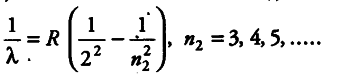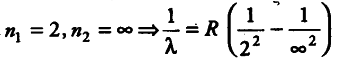# Calculate the shortest wavelength in the Balmer series of hydrogen atom

Calculate the shortest wavelength in the Balmer series of hydrogen atom. In which region (infrared, visible, ultraviolet) of hydrogen spectrum does this wavelength lie?

Since, we know that for Balmer series,For shortest wavelength in Balmer series, the spectral series is given by1/\lambda = R x 1/4
1/\lambda = R/4
\lambda = 4/R
\lambda = 4 / 1.097 x {{10}^{7}}
\lambda = 3.64 x {{10}^{-7}} m
The lines of Balmer series are found in the visible part of the spectrum.